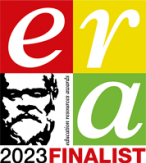# AQA GCSE (8525) SLR 13 – Data representation

This download provides you with a zip file which contains all the resources you will need to deliver this topic.

It includes:

• Student Learning Record Workbook (for use by students)
• Student Learning Record Answers (for use by teachers)
• End of Topic test and answers
• Lesson PowerPoint’s for every lesson
• Any associated answers or references sheets for class activities

This topic covers:

• 3.3.1 – 3.3.8 from the specification (8525)

3.3.1 Number bases

• Understand the following number bases:
decimal (base 10)
binary (base 2)
• Understand that computers use binary to represent all data and instructions.
• Explain why hexadecimal is often used in computer science.

3.3.2 Converting between number bases

• Understand how binary can be used to represent whole numbers.
• Understand how hexadecimal can be used to represent whole numbers.
• Be able to convert in both directions between:
binary and decimal

3.3.3 Units of information

• Know that:
a bit is the fundamental unit of information
a byte is a group of 8 bits.
• Know that quantities of bytes can be described using prefixes.
• Know the names, symbols and corresponding values for the decimal prefixes:
kilo, 1 kB is 1,000 bytes
mega, 1 MB is 1,000 kilobytes
giga, 1 GB is 1,000 Megabytes
tera, 1 TB is 1,000 Gigabytes
• Be able to compare quantities of bytes using the prefixes above.

3.3.4 Binary arithmetic

• Be able to add together up to three binary numbers.
• Be able to apply a binary shift to a binary number.
• Describe situations where binary shifts can be used.

3.3.5 Character encoding

• Understand what a character set is and be able to describe the following character encoding methods:
7-bit ASCII
Unicode
• Understand that character codes are commonly grouped and run in sequence within encoding tables.
• Describe the purpose of Unicode and the advantages of Unicode over ASCII.
• Know that Unicode uses the same codes as ASCII up to 127.

3.3.6 Representing images

• Understand what a pixel is and be able to describe how pixels relate to an image and the way images are displayed.
• Describe the following for bitmaps:
image size
colour depth
• Know that the size of a bitmap image is measured in pixels (width x height).
• Describe how a bitmap represents an image using pixels and colour depth.
• Describe using examples how the number of pixels and colour depth can affect the file size of a bitmap image.
• Calculate bitmap image file sizes based on the number of pixels and colour depth.
• Convert binary data into a bitmap image.
• Convert a bitmap image into binary data.

3.3.7 Representing sound

• Understand that sound is analogue and that it must be converted to a digital form for storage and processing in a computer.
• Understand that analogue signals are sampled to create the digital version of sound.
• Describe the digital representation of sound in terms of:
sampling rate
sample resolution
• Calculate sound file sizes based on the sampling rate and the sample resolution.

3.3.8 Data compression

• Explain what data compression is.
• Understand why data may be compressed and that there are different ways to compress data.
• Explain how data can be compressed using Huffman coding.
• Be able to interpret Huffman trees.
• Be able to calculate the number of bits required to store a piece of data compressed using Huffman coding.
• Be able to calculate the number of bits required to store a piece of uncompressed data in ASCII.
• Explain how data can be compressed using run length encoding (RLE).
• Represent data in RLE frequency/data pairs.Registered in England and Wales: 10442992

VAT Number: 290 9845 58

Telephone: 020 4519 3010